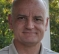## How to manipulate a spectrum without aliasing problems?Started by 5 years ago7 replieslatest reply 5 years ago218 views

Hi everyone.

For various tasks in my day-to-day job I run into the same problem. I would like to do the following:

- Slice a one dimensional signal x into chunks of N samples and transform them into the frequency domain (FFT/MDCT, what ever).

- modify the single bins in the frequency domain (attenuate noisy bins or perform echo-cancelling or so).

- transform back to time domain

The "modify" step can be expressed as creating a mask of N gains (call it M) and multiplying the signal spectrum with it. This is equivalent to a (circular) convolution of the time-domain signals. This convolution - depending on the shape of M - will lead to a signal of max length 2*N-1. Since the convolution is circular, it will wrap around at the chunk ends and create aliasing.

In standard fast convolution I would zero-pad both operands to length 2*N before transforming into the frequency domain, multiplying and inverse-transforming. I could overlap and add the chunks and I will get no aliasing problems. But since I modify bins in the frequency domain I cannot do this to the modification mask M.

I can think of these (equivalent) solutions:

First:

- zero-pad x and transform to f-domain (length is 2*N)
- create modification mask M (length 2*N) and transform M back to time domain (m).
- truncate m at N samples, zero-pad to 2N and transform back to frequency domain (M)
- multiply M and X, transform back, overlap and add and we're done

Second:

- zero-pad x and transform to f-domain (length is 2*N)
- create modification mask M (length 2*N).
- convolute M with sinc (transformed time-domain rect with length N) in the f-domain
- multiply M and X, transform back, overlap and add and we're done

Both solutions create an alias-free output signal.
Both solutions limit the resolution of M - which is not nice, but I could live with it.
Both solutions eliminate the advantage of being able to just mess with the frequency bins and be done - it's just so much more calculation effort that it can make a time-domain solution seem more attractive.

QUESTION:

Are there any other ways to deal with this problem?

Is there a transform that compensates for this?

Is there a quicker way to eliminate the aliases than what I described above?

[ - ]For cancellation, and noise reduction, the most common frequency domain techniques use FFTs, but usually as part of a sub-band processing scheme using filter-banks.  These also involve an overlap-add component as well.

Have you looked at filter-banks as a complete solution?

If the polyphase filters are carefully designed, then the aliasing components can be completely compensated (E.G. Quadrature mirror filters).  However, in my experience, the improvement in performance achieved by using an "ideal alias-free filter-bank" is un-warranted.  Most of the time a very good compromise can be found, and often at lower complexity.

[ - ]I have used QMFs for a low-latency audio compression I designed a few years ago - I haven't considered them for my current task for some reason. Thanks for the hint.

In your opinion, would using the MCLT (modulated complex lapped tranform) cancel the kind of aliasing described above or am I mislead there...

[ - ]The 3gpp standard for wide-band speech coders, has an example C code reference that includes the full sub-band separation for the noise reduction algorithm.  This might be a useful reference.

The specification for EVRC is available at the following link.

See section 4.4.3. for the noise suppression algorithm.

[ - ]Unfortunately, am not familiar with MCLT, so can't comment on their use here.

Thanks for the reference though.

[ - ]Are you actively deciding on what operations to do in the frequency domain each time, or are you making decisions on a per-batch basis, or are you always doing the same operations to the same bins day in and day out?

Because a light reading of your description is just overlap-and-add filtering, and a deeper reading doesn't tell me what you're trying to accomplish.

If you are just applying fixed filtering, then pre-compute the FIR you need to do to accomplish it, and apply it.

[ - ]In my opinion this could work to get an alias free signal:

- zero-pad x (to length 2*N) and transform to f-domain

(N+1 frequency bins = N complex bins + DC)

- create modification mask M (length N+1 including the DC-component)

- multiply M and X, transform back, truncate to N points, overlap and add

HP

[ - ]